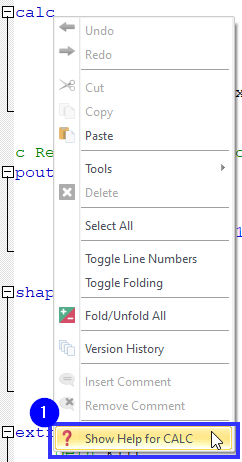# how to plot the ricker wavelet in Matlab？ Answered

Onscale and pzlex can code the function of Ricker wavelet with using wvlt command.I wanna know how to plot the ricker wavelet in Matlab and output the data?

• The Ricker Wavelet isn't unique to OnScale but have a look at this: https://uk.mathworks.com/help/wavelet/ref/mexihat.html

This is a forum for OnScale and PZFlex issues. If you want to do this in MATLAB or have any more issues with this in MATLAB then this is a question that should be asked on MATLAB forums.

• Thanks a lot.

And another question,is there any example of stree computed in the Analyst ？

• There will be certain examples that calculate and output stress and strain.

If you open the documentation and look at the CALC STRS and STRN command it will provide more information